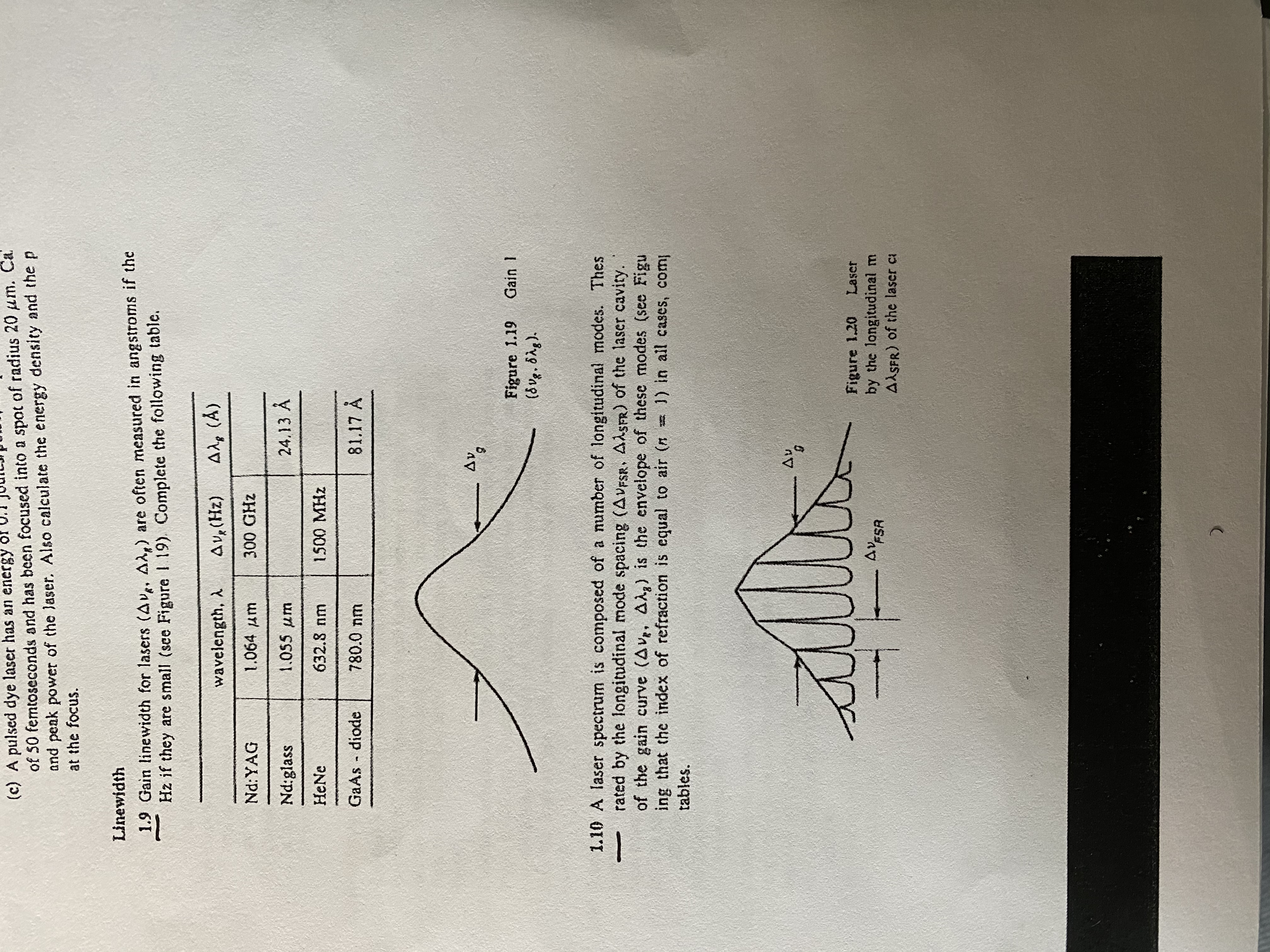# (c) A pulsed dye laser has an energy of 50 femtoseconds and has been focused into a spot of radius 20 um. Ca and peak power of the laser. Also calculate the energy density and the p at the focus. Linewidth 1.9 Gain linewidth for lasers (Av.. Ad,) are often measured in angstroms if the Hz if they are small (sce Figure I 19). Complete the following table. Ale (A) AVe (Hz) wavelength, A 1.064 am 300 GHz Nd:YAG 24.13 A 1.055 um Nd:glass 632.8 nm HeNe 1500MHZ GaAs diode 81.17 A 780.0 nm Figure 1.19 (d ug. 8Ag). Gain 1 1.10 A laser spectrum is composed of a number of longitudinal modes. Thes rated by the longitudinal mode spacing (AVFSR, AASFR) of the laser cavity. of the gain curve (Av, Alg) is the envelope of these modes (see Figu ing that the index of refraction is equal to air (n 1) in all cases, com tables. Av Figure 1.20 by the longitudinal m AASFR) of the laser ca Laser AVFSR

Question

1.9help_outlineImage Transcriptionclose(c) A pulsed dye laser has an energy of 50 femtoseconds and has been focused into a spot of radius 20 um. Ca and peak power of the laser. Also calculate the energy density and the p at the focus. Linewidth 1.9 Gain linewidth for lasers (Av.. Ad,) are often measured in angstroms if the Hz if they are small (sce Figure I 19). Complete the following table. Ale (A) AVe (Hz) wavelength, A 1.064 am 300 GHz Nd:YAG 24.13 A 1.055 um Nd:glass 632.8 nm HeNe 1500MHZ GaAs diode 81.17 A 780.0 nm Figure 1.19 (d ug. 8Ag). Gain 1 1.10 A laser spectrum is composed of a number of longitudinal modes. Thes rated by the longitudinal mode spacing (AVFSR, AASFR) of the laser cavity. of the gain curve (Av, Alg) is the envelope of these modes (see Figu ing that the index of refraction is equal to air (n 1) in all cases, com tables. Av Figure 1.20 by the longitudinal m AASFR) of the laser ca Laser AVFSR fullscreen